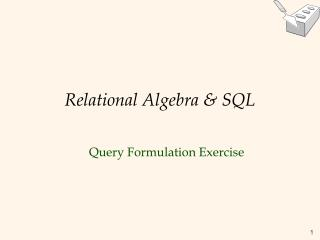DownloadDownload PresentationRelational Algebra & SQL

# Relational Algebra & SQL

Download Presentation## Relational Algebra & SQL

- - - - - - - - - - - - - - - - - - - - - - - - - - - E N D - - - - - - - - - - - - - - - - - - - - - - - - - - -
##### Presentation Transcript

1. Relational Algebra & SQL Query Formulation Exercise

2. Exercise 4: Relational Algebra and SQL Given relational schema: Frequent (D, P) Serves (P, B) Likes (D, B) Attributes: P (pub), B (beer), D (drinker) • The pubs that serve a beer that Jefferson likes. • Drinkers that frequent at least one pub that serves “Bud” or “Becks”. • Drinkers that frequent only pubs that serve some beer they like • Drinkers that frequent only pubs that serve no beer they like.

3. 1) Algebra • Solution 1: • Solution 2: • Solution 3: • Solution 4:

4. 2) SQL SELECT p FROM S, L WHERE S.b=L.b AND L.d=‘Jefferson’ • Solution 1: • Solution 2: SELECT d FROM F WHERE F.p in (SELECT p FROM S WHERE b IN (‘Bud”, ‘Becks’))

5. 2) SQL SELECT d FROM F EXCEPT SELECT d FROM (SELECT* FROM F EXCEPT SELECT d,p FROM S, L WHERE S.b = L.b) • Solution 3:

6. 2) SQL SELECT d FROM F EXCEPT SELECT d FROM (SELECT* FROM F INTERSECT SELECT d,p FROM S, L WHERE S.b = L.b) • Solution 4:

7. 2) SQL SELECT d FROM F WHERE (SELECT COUNT (DISTINCT p) FROM S, L WHERE F.p=S.p AND S.b=L.b AND L.d=F.d) = 0 • Solution 4 (alternative solution):